Next: Formulation of the statistical Up: Quantum statistics Previous: Symmetry requirements in quantum

## An illustrative example

Consider a very simple gas made up of two identical particles. Suppose that each particle can be in one of three possible quantum states,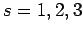. Let us enumerate the possible states of the whole gas according to Maxwell-Boltzmann, Bose-Einstein, and Fermi-Dirac statistics, respectively.

For the case of Maxwell-Boltzmann (MB) statistics, the two particles are considered to be distinguishable. Let us denote themand. Furthermore, any number of particles can occupy the same quantum state. The possible different states of the gas are shown in Tab. 6.

Table 6: Two particles distributed amongst three states according to Maxwell-Boltzmann statistics.
 1 2 3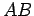There are clearly 9 distinct states.

For the case of Bose-Einstein (BE) statistics, the two particles are considered to be indistinguishable. Let us denote them both as. Furthermore, any number of particles can occupy the same quantum state. The possible different states of the gas are shown in Tab. 7.

Table 7: Two particles distributed amongst three states according to Bose-Einstein statistics.
 1 2 3There are clearly 6 distinct states.

Finally, for the case of Fermi-Dirac (FD) statistics, the two particles are considered to be indistinguishable. Let us again denote them both as. Furthermore, no more than one particle can occupy a given quantum state. The possible different states of the gas are shown in Tab. 8.

Table 8: Two particles distributed amongst three states according to Fermi-Dirac statistics.
 1 2 3There are clearly only 3 distinct states.

It follows, from the above example, that Fermi-Dirac statistics are more restrictive (i.e., there are less possible states of the system) than Bose-Einstein statistics, which are, in turn, more restrictive than Maxwell-Boltzmann statistics. Let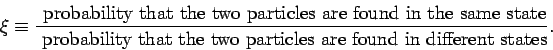(575)

For the case under investigation,(576)(577)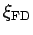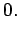(578)

We conclude that in Bose-Einstein statistics there is a greater relative tendency for particles to cluster in the same state than in classical statistics. On the other hand, in Fermi-Dirac statistics there is less tendency for particles to cluster in the same state than in classical statistics.Next: Formulation of the statistical Up: Quantum statistics Previous: Symmetry requirements in quantum
Richard Fitzpatrick 2006-02-02